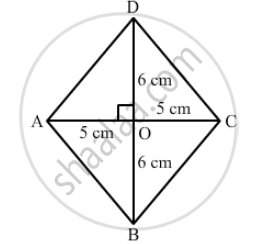Advertisement Remove all ads

# If the Diagonals of a Rhombus Are 12 Cm and 16cm, Find the Length of Each Side. - Mathematics

If the diagonals of a rhombus are 12 cm and 16cm, find the length of each side.

Advertisement Remove all ads

#### Solution$\text{ All sides of a rhombus are equal in length }.$
$\text{ The diagonals intersect at 90\textdegree and the sides of the rhombus form right triangles } .$
$\text{ One leg of these right triangles is equal to 8 cm and the other is equal to 6 cm } .$
$\text{ The sides of the triangle form the hypotenuse of these right triangles }.$
$\text{ So, we get }:$
$( 8^2 + 6^2 ) {cm}^2$
$= (64 + 36) {cm}^2$
$= 100 {cm}^2$
$\text{ The hypotneuse is the square root of 100 {cm}^2 . This makes the hypotneuse equal to 10 }.$
$\text{ Thus, the side of the rhombus is equal to 10 cm } .$

Is there an error in this question or solution?
Advertisement Remove all ads

#### APPEARS IN

RD Sharma Class 8 Maths
Chapter 17 Understanding Shapes-III (Special Types of Quadrilaterals)
Exercise 17.2 | Q 6 | Page 17
Advertisement Remove all ads

#### Video TutorialsVIEW ALL 

Advertisement Remove all ads
Share
Notifications

View all notifications

Forgot password?
Course